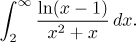# Solved Problems for Improper Integrals

If you wish to simultaneously follow another text on improper integrals in a separate window, click here for Theory and here for Methods Survey.

• Problem: Evaluate (if it converges) the integral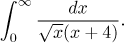• Problem: Evaluate (if it converges) the integral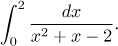• Problem: Evaluate (if it converges) the integral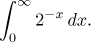• Problem: Decide whether the following integral converges: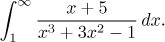• Problem: Decide whether the following integral converges: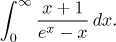• Problem: Decide whether the following integral converges: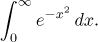• Problem: Decide whether the following integral converges: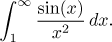• Problem: Decide whether the following integral converges: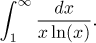• Problem: Decide whether the following integral converges: